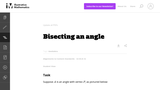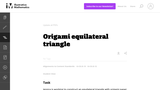# 18 Results

View
Selected filters:
• WI.Math.Content.HSG-CO.D.12Conditional Remix & Share Permitted
CC BY-NC-SA
Rating

This is a task from the Illustrative Mathematics website that is one part of a complete illustration of the standard to which it is aligned. Each task has at least one solution and some commentary that addresses important asects of the task and its potential use. Here are the first few lines of the commentary for this task: This task examines the mathematics behind an origami construction of a rectangle whose sides have the ratio $(\sqrt{2}:1)$. Such a rectangle is called ...

Subject:
Mathematics
Material Type:
Activity/Lab
Provider:
Illustrative Mathematics
Provider Set:
Illustrative Mathematics
Author:
Illustrative Mathematics
10/14/2013Conditional Remix & Share Permitted
CC BY-NC-SA
Rating

This task provides a construction of the angle bisector of an angle by reducing it to the bisection of an angle to finding the midpoint of a line segment. It is worth observing the symmetry -- for both finding midpoints and bisecting angles, the goal is to cut an object into two equal parts. The conclusion of this task is that they are, in a sense, of exactly equivalent difficulty -- bisecting a segment allows us to bisect and angle (part a) and, conversely, bisecting an angle allows us to bisect a segment (part b). In addition to seeing how these two constructions are related, the task also provides an opportunity for students to use two different triangle congruence criteria: SSS and SAS.

Subject:
Mathematics
Geometry
Material Type:
Activity/Lab
Provider:
Illustrative Mathematics
Provider Set:
Illustrative Mathematics
Author:
Illustrative Mathematics
01/11/2013Conditional Remix & Share Permitted
CC BY-NC-SA
Rating

This task provides the most famous construction to bisect a given angle. It applies when the angle is not 180 degrees.

Subject:
Mathematics
Geometry
Material Type:
Activity/Lab
Provider:
Illustrative Mathematics
Provider Set:
Illustrative Mathematics
Author:
Illustrative Mathematics
01/11/2013
RemixConditional Remix & Share Permitted
CC BY-NC-SA
Rating

Students will collaboratively design and construct a cardboard boat using a variety of tools and methods which will float and hold 1 human in the school pool.

Subject:
Education
Character Education
Speaking and Listening
Geometry
Measurement and Data
Material Type:
Lesson
Lesson Plan
Author:
Blake Jersey
Lynn Aprill
09/16/2019
RemixConditional Remix & Share Permitted
CC BY-NC-SA
Rating

Students will collaboratively design and construct a cardboard boat using a variety of tools and methods which will float and hold 1 human in the school pool.

Subject:
Education
Character Education
Speaking and Listening
Geometry
Measurement and Data
Material Type:
Lesson
Lesson Plan
Author:
Blake Jersey
08/21/2019
RemixConditional Remix & Share Permitted
CC BY-NC-SA
Rating

Students will collaboratively design and construct a cardboard boat using a variety of tools and methods which will float and hold 1 human in the school pool.

Subject:
Education
Character Education
Speaking and Listening
Geometry
Measurement and Data
Material Type:
Lesson
Lesson Plan
Author:
Blake Jersey
09/16/2019Conditional Remix & Share Permitted
CC BY-NC-SA
Rating

The construction of the perpendicular bisector of a line segment is one of the most common in plane geometry and it is undertaken here. In addition to giving students a chance to work with straightedge and compass, the problem uses triangle congruence both to show that the constructed line is perpendicular to AB and to show that it bisects AB.

Subject:
Mathematics
Geometry
Material Type:
Activity/Lab
Provider:
Illustrative Mathematics
Provider Set:
Illustrative Mathematics
Author:
Illustrative Mathematics
01/11/2013Conditional Remix & Share Permitted
CC BY-NC-SA
Rating

This is a task from the Illustrative Mathematics website that is one part of a complete illustration of the standard to which it is aligned. Each task has at least one solution and some commentary that addresses important asects of the task and its potential use. Here are the first few lines of the commentary for this task: Jessica is working to construct an equilateral triangle with origami paper and uses the following steps. First she folds the paper in half and then unf...

Subject:
Mathematics
Material Type:
Activity/Lab
Provider:
Illustrative Mathematics
Provider Set:
Illustrative Mathematics
Author:
Illustrative Mathematics
10/14/2013Conditional Remix & Share Permitted
CC BY-NC-SA
Rating

This is a task from the Illustrative Mathematics website that is one part of a complete illustration of the standard to which it is aligned. Each task has at least one solution and some commentary that addresses important asects of the task and its potential use. Here are the first few lines of the commentary for this task: Lisa makes an octagon by successively folding a square piece of paper as follows. First, she folds the square in half vertically and horizontally and a...

Subject:
Mathematics
Material Type:
Activity/Lab
Provider:
Illustrative Mathematics
Provider Set:
Illustrative Mathematics
Author:
Illustrative Mathematics
10/14/2013Conditional Remix & Share Permitted
CC BY-NC-SA
Rating

Module 1 embodies critical changes in Geometry as outlined by the Common Core. The heart of the module is the study of transformations and the role transformations play in defining congruence. The topic of transformations is introduced in a primarily experiential manner in Grade 8 and is formalized in Grade 10 with the use of precise language. The need for clear use of language is emphasized through vocabulary, the process of writing steps to perform constructions, and ultimately as part of the proof-writing process.

Subject:
Geometry
Material Type:
Module
Provider:
New York State Education Department
Provider Set:
EngageNY
05/14/2013Conditional Remix & Share Permitted
CC BY-NC-SA
Rating

Understanding vocabulary is critical in order to fully understand triangle relationships. In this activity, students will review the vocabulary associated with triangles: circumcenter, incenter, centroid, and orthocenter.

I had my students cut out the flashcards during class. I was very surprised some students took a lot of time cutting out the cards since they cut around the boxes instead of making straight cuts.

I would suggest before students are given the scissors, to model the quickest way to cut out the cards.

Subject:
Mathematics
Material Type:
Activity/Lab
Author:
Linda Gregory
08/02/2019Educational Use
Rating

Students learn about common geometry tools and then learn to use protractors (and Miras, if available) to create and measure angles and reflections. The lesson begins with a recap of the history and modern-day use of protractors, compasses and mirrors. After seeing some class practice problems and completing a set of worksheet-prompted problems, students share their methods and work. Through the lesson, students gain an awareness of the pervasive use of angles, and these tools, for design purposes related to engineering and everyday uses. This lesson prepares students to conduct the associated activity in which they “solve the holes” for hole-in-one multiple-banked angle solutions, make their own one-hole mini-golf courses with their own geometry-based problems and solutions, and then compare their “on paper” solutions to real-world results.

Subject:
Mathematics
Geometry
Material Type:
Lesson
Provider:
TeachEngineering
Author:
Aaron Lamplugh
Andi Vicksman
CU Teach Engineering (a STEM licensure pathway), Engineering Plus Degree Program, University of Colorado Boulder
Devin Rourke
Malinda Zarske
Nathan Coyle
Russell Anderson
Ryan Sullivan
10/13/2017Conditional Remix & Share Permitted
CC BY-NC-SA
Rating

This task can be implemented in a variety of ways. For a class with previous exposure to the incenter or angle bisectors, part (a) could be a quick exercise in geometric constructions,. Alternatively, this could be part of a full introduction to angle bisectors, culminating in a full proof that the three angle bisectors are concurrent, an essentially complete proof of which is found in the solution below.

Subject:
Mathematics
Geometry
Material Type:
Activity/Lab
Provider:
Illustrative Mathematics
Provider Set:
Illustrative Mathematics
Author:
Illustrative Mathematics
05/01/2012Conditional Remix & Share Permitted
CC BY-NC-SA
Rating

This task can be implemented in a variety of ways. For a class with previous exposure to properties of perpendicular bisectors, part (a) could be a quick exercise in geometric constructions, and an application of the result. Alternatively, this could be part of an introduction to perpendicular bisectors, culminating in a full proof that the three perpendicular bisectors are concurrent at the circumcenter of the triangle, an essentially complete proof of which is found in the solution below.

Subject:
Mathematics
Geometry
Material Type:
Activity/Lab
Provider:
Illustrative Mathematics
Provider Set:
Illustrative Mathematics
Author:
Illustrative Mathematics
05/01/2012Unrestricted Use
CC BY
Rating

This is a high school geometry task that has students physically construct the point equidistant from three non-collinear points and to identify why the construction works.  This construction motivates the notion of a triangle inscribed into a circle and why that particular construction might be useful.

This task is a procedures with connections task, of high cognitive demand.  The procedure is not specified for students but there is largely only one way of folding the paper to be able to identify the intersection point.  The high cognitive demand comes from students having to explain why the construction works and why only two creases are necessary.  This gets at both the meaning and motivation for the construction and the notion of efficiency in having a canonical construction for a circle that inscribes a triangle given three non-collinear points that can form a triangle.

This task could also be used as an assessment task after students learn the construction, although the explanations that may be given by students are more likely to focus on the construction procedures in this particular case.

This task addresses the Pivotal Understanding of equivalence, because it focuses on generating a geometric construction procedure that determines a point equidistant from three non-collinear points.  Equivalence is evident in at least two ways.  First, the distance from the target point to each of the source points is equal.  Second, the construction produces equivalent results (inscribed triangle within a circle given three points) each time.

Subject:
Mathematics
Material Type:
Assessment Item
Formative Assessment
Provider:
Illustrative Mathematics
12/21/2015Conditional Remix & Share Permitted
CC BY-NC
Rating

In this activity, students will construct using a compass and straightedge the points of concurrency in triangles: centroid, incenter/incircle, circumcenter/circumcircle, and the orthocenter.

Subject:
Mathematics
Material Type:
Activity/Lab
Author:
Linda Gregory
08/02/2019Conditional Remix & Share Permitted
CC BY-NC-SA
Rating

This task is a reasonably straight-forward application of rigid motion geometry, with emphasis on ruler and straightedge constructions, and would be suitable for assessment purposes.

Subject:
Mathematics
Geometry
Material Type:
Activity/Lab
Provider:
Illustrative Mathematics
Provider Set:
Illustrative Mathematics
Author:
Illustrative Mathematics
05/01/2012Rating

Students construct "a tetrahedron and describe the linear, area and volume using non-traditional units of measure. Four tetrahedra are combined to form a similar tetrahedron whose linear dimensions are twice the original tetrahedron. The area and volume relationships between the first and second tetrahedra are explored, and generalizations for the relationships are developed." (from NCTM Illuminations)

Subject:
Mathematics
Geometry
Material Type:
Interactive
Lesson Plan
Provider:
National Council of Teachers of Mathematics
Provider Set:
Illuminations
Author:
Illuminations National Council of Teachers of Mathematics
Kris A. Warloe
NCTM Illuminations
Thinkfinity/Verizon Foundation